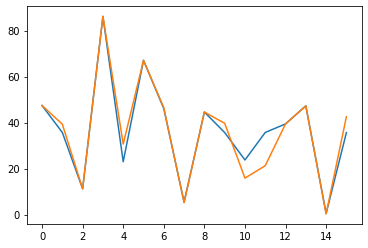# Linear regression loss function value does not decrease

Hi everyone!
I’m pretty new to Machine Learning and I was trying to implement Linear Regression to predict house prices.
The issue that I’m facing is that during training, my loss function does not decrease or it decreases just a bit. It’s stuck on value `165283216.0` which is not satisfying at all…

Here’s the code:

``````# house_features is pandas dataframe with columns: 'district', 'rooms', 'square_meters'
# house_prices is a dataframe with just one column: 'price'
X_train, x_test, Y_train, y_test = train_test_split(house_features, house_prices, test_size=0.2, random_state=42)

# Here I'm converting data to tensors
dtype = torch.float
X_train_tensor = torch.tensor(X_train.values, dtype=dtype)
x_test_tensor = torch.tensor(x_test.values, dtype=dtype)

Y_train_tensor = torch.tensor(Y_train.values, dtype=dtype)
y_test_tensor = torch.tensor(y_test.values, dtype=dtype)

# Configuration values
input_features_amount = 3
output_amount = 1
hidden_layer_size = 10

loss_function = torch.nn.MSELoss()
learning_rate = 1e-4

model = torch.nn.Sequential(torch.nn.Linear(input_features_amount, hidden_layer_size),
torch.nn.Sigmoid(),
torch.nn.Linear(hidden_layer_size, output_amount))

loss_list = []
EPOCHS = 10_000
# Here I'm reshaping tensor so that the shapes would match - I was receiving an error without this line
Y_train_tensor = torch.reshape(Y_train_tensor, (X_train_tensor.shape, 1))

for epoch in range(EPOCHS):
y_pred = model(X_train_tensor)
loss = loss_function(y_pred, Y_train_tensor)

if epoch % 1000 == 0:
print(epoch, loss.item())

loss_list.append(loss.item())
loss.backward()

for param in model.parameters():
``````

I attach also a screenshot from Jupyter Notebook with training loss function values. Does anyone know what might be the reason of this issue?
I went through a couple of similar posts but I wasn’t able to implement any reasonable fix.

About dataset, I built it myself by scrapping some page with house rental offers and it consists of ~1000 records.

Did you scale the features in any way? Usually neural networks won’t learn well if the features are not scaled.

I didn’t before, but now I used Standarization:

``````scaler = StandardScaler()
X_train_norm = scaler.fit_transform(X_train)
``````

It got a little bit better, but the result is still not satisfyingI can see now that you also unnecesserily do the parameters update manually at the end of the code. Have a look at pytorch tutorials to see how to contruct proper training loop.

Your current code works ~alright for overfitting random data:

``````# Configuration values
input_features_amount = 3
output_amount = 1
hidden_layer_size = 10

X_train_tensor = torch.randn(16, input_features_amount)
Y_train_tensor = torch.rand(16, 1) * 100

loss_function = torch.nn.MSELoss()
learning_rate = 1e-3

model = torch.nn.Sequential(torch.nn.Linear(input_features_amount, hidden_layer_size),
torch.nn.Sigmoid(),
torch.nn.Linear(hidden_layer_size, output_amount))

loss_list = []
EPOCHS = 100_000

for epoch in range(EPOCHS):
y_pred = model(X_train_tensor)
loss = loss_function(y_pred, Y_train_tensor)

if epoch % 1000 == 0:
print(epoch, loss.item())

loss_list.append(loss.item())
loss.backward()Using `nn.ReLU` instead of `nn.Sigmoid` seems to help and indeed using a built-in optimizer, as suggested by @The_Snail, might help.